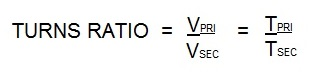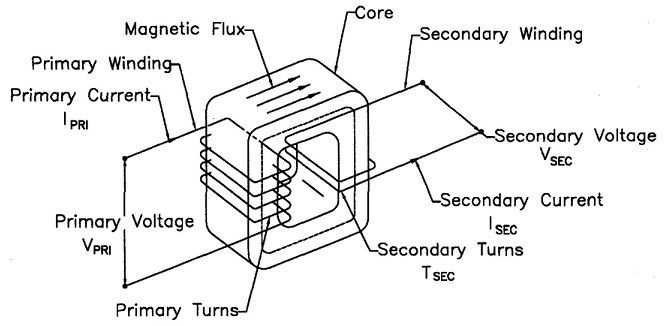# Do I need to install a series bar to measure the turns ratio of a resistance welding transformer?

The transformer turns ratio in resistance welding reflects the number of secondary coil turns versus the number of primary coil turns. In AC transformers, there are many turns in the primary. The secondary has one turn. If there are 50 turns then the ratio is 50/1. Therefor the primary voltage will drop from 220 V and 100 amp input to 220/50 = 4 volts in the secondary. The equations for this are:Vpri = Voltage on the primary                Tpri = Turns in the primary coil

Vsec= Voltage on the secondary          Tsec = Turns in the secondary coilPower into Transformer equals power out of Transformer

Frequently transformers have tap switches or are built with parallel circuits. In this case a series bar can be installed to change the turns ratio or the tap can be changed to a different turns ratio.
To measure the actual turns ratio one must take the input output readings as shown above for the different taps or with the bar in or out to learn which is the turns ratio desired. This will depend upon the type of transformer you have.
Of course first look at the transformer drawing which will show the schematic with this information included.

Another article in this blog on this subject is:

WHAT IS TRANSFORMER TURNS RATIO FOR A SPOT WELDER?

Reference: RWMA Resistance Welding Manual, Chapter 19

### Have a Question?

Do you have a question that is not covered in our knowledgebase? Do you have questions regarding the above article? Click here to ask the professor.